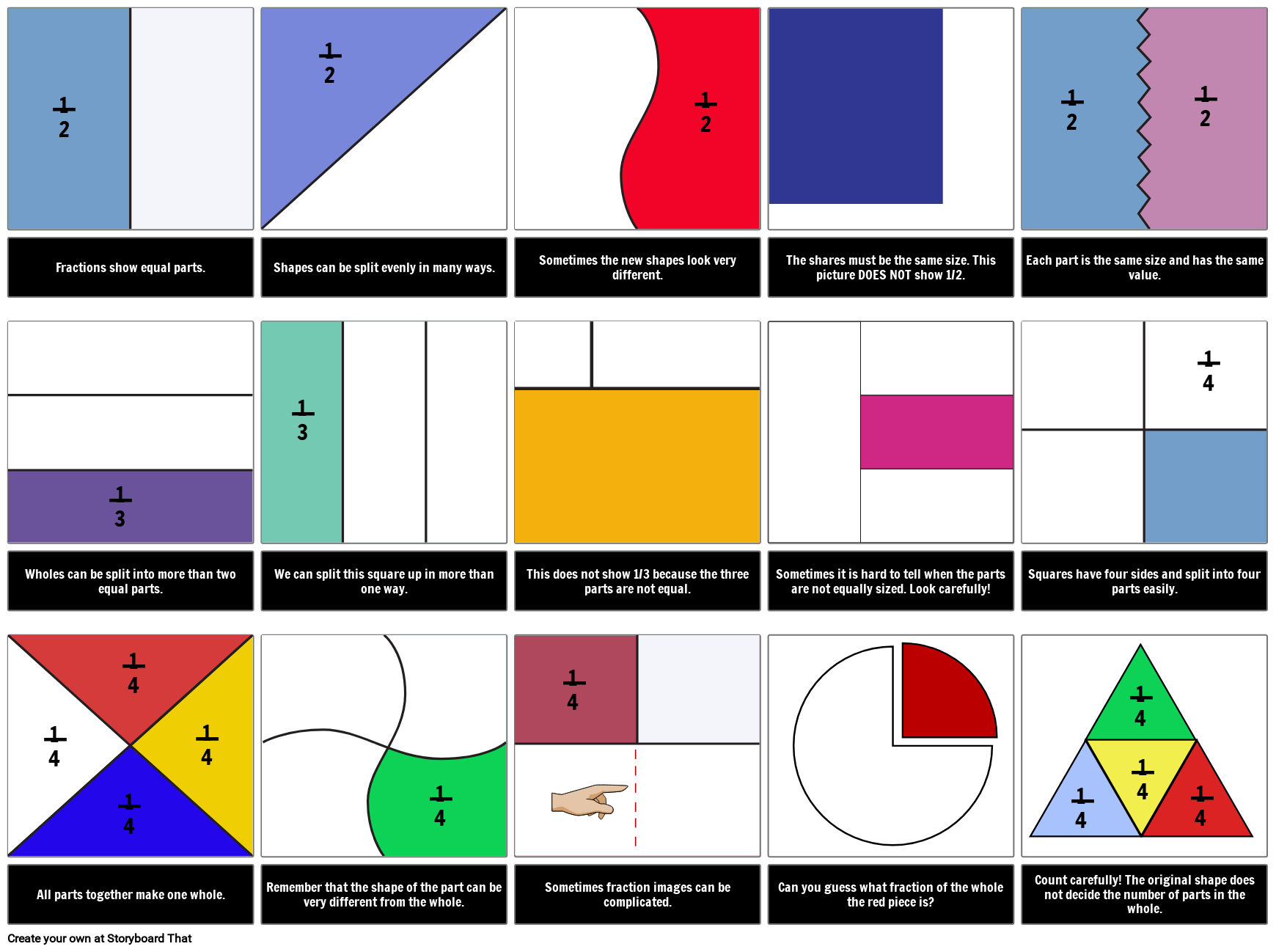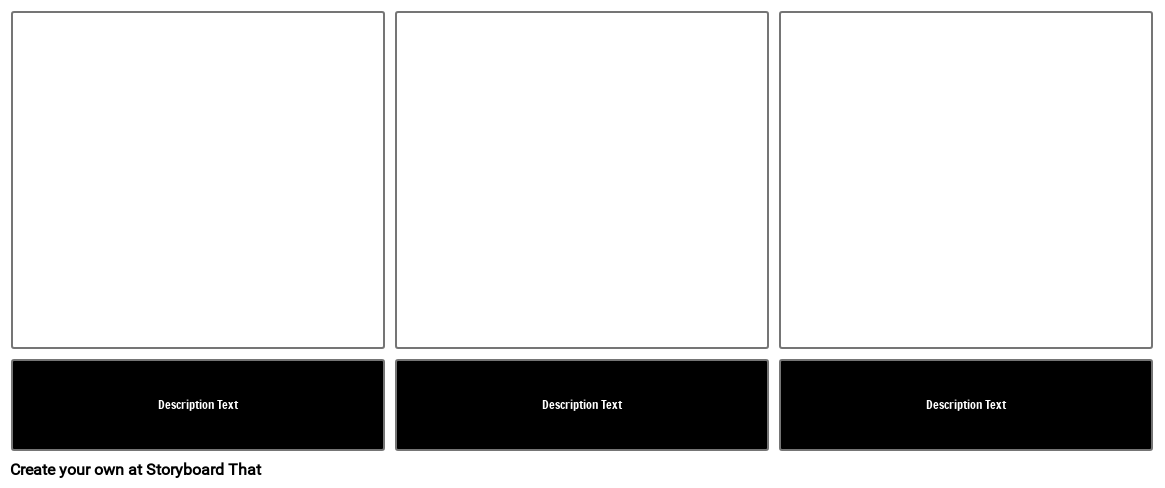## Activity Overview

When students are learning about fractions, a visual aid can be extremely helpful. Fractions show equal shares. Pictures that show shapes partitioned into sections that are not the same size do not show examples of fractions. In this activity, you'll show pictures of shapes that have been equally and unequally divided. In this activity, students will identify whether something is a fraction and then determine what the fraction is.

Allow students to identify the total number of parts in a whole, such as a circle, to find the denominator. The bottom of the fraction, represents the number of partitions (equal shares). Each piece of the whole is therefore one of the whole. Have students guess what to call each part (one half, one third, one fourth etc.) and create a chart together to show the first few common unit fractions.

The storyboard above gives you a great visual aid to use as part of a presentation, and you can turn it into an assignment for students by altering the example and adding to the assignment. The instructions for this are included below. Students can also create their own instructional comic about fractions as an assessment of understanding.

## Template and Class Instructions

(These instructions are completely customizable. After clicking "Use This Assignment With My Students", update the instructions on the Edit Tab of the assignment.)

Student Instructions

Identify whether the image shows a fraction (shows equal shares) and if so, identify the fractions that make up the shape.

1. Click "Start Assignment".
2. Identify whether the shape shown is an example of a fraction.
3. If it is, use a textable to label the fraction of the colored section.
4. Save and submit your storyboard.

#### Lesson Plan Reference

Difficulty Level 3 (Developing to Mastery)

Type of Assignment Individual or Partner

Common Core Standards
• [Math/Content/3/G/A/2] Partition shapes into parts with equal areas. Express the area of each part as a unit fraction of the whole. For example, partition a shape into 4 parts with equal area, and describe the area of each part as 1/4 of the area of the shape.## More Storyboard That Activities

### Introduction to Fractions

*(This will start a 2-Week Free Trial - No Credit Card Needed)